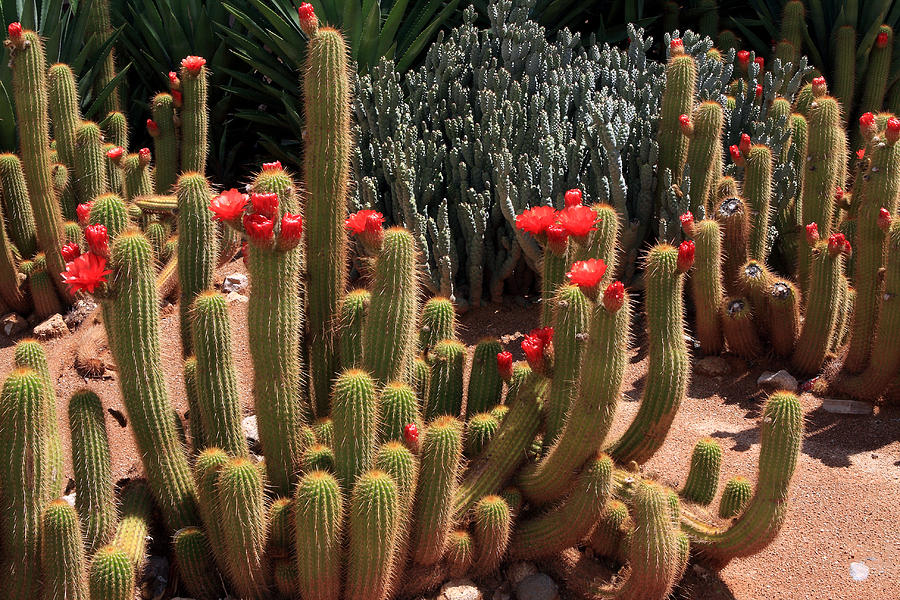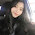## Thursday, 17 January 2019

### SLJ - Day 2 - Activity 3

Activity 3: On your blog, tell us how to solve this maths problem. You can write your answer in words, use a Sketchpad image, or post a video explaining how you would figure it out. Be sure to give us your final answer in millilitres (ml).

Work:3 x 365 = ??
{breaking it down so it can be easier}

3 x 5 = 15
3 x 60 = || 3 x 0 = 0 || 3 x 6 = 18 || add the 0 to the 18 and you get 180
3 x 300 = || 3 x 0 = 0 || 3 x 0 = 0 || 3 x 3 = 9 || Add the two 0's to the 9 and you get 900
15 + 180 + 900 = 1095

#### 1 comment:

1.Hi Francesca,

Congratulations on having a go at solving this maths problem. I like how you have carefully thought about the place value to figure out what each number actually represents in '365'. As you've mentioned, this number basically consists of 300, 60 and 5.

It was indeed an easier way to solve this multiplication question so ka pai for coming up with the strategy! Do you enjoy maths at school? If not, what is your favourite subject?

Keep up the awesome work,
Susie :)